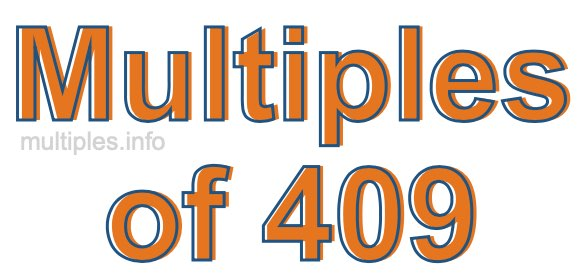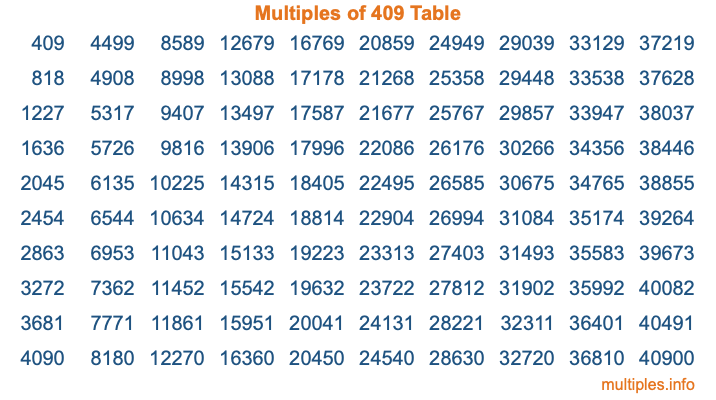Multiples of 409Welcome to the Multiples of 409 page. Here we will first teach you everything you will ever need to know about the multiples of 409, and then give you a study guide summary of everything we taught you to make sure you remember it all. Use this page to look up facts and learn information about the multiples of 409. This page will make you a multiples of four hundred nine expert!

Definition of Multiples of 409
Multiples of 409 are all the numbers that when divided by 409 equal an integer. Each of the multiples of 409 are called a multiple. A multiple of 409 is created by multiplying 409 by an integer.

Therefore, to create a list of multiples of 409, you start with 1 multiplied by 409, then 2 multiplied by 409, then 3 multiplied by 409, and so on for as long as you want. Thus, the list of the first five multiples of 409 is 409, 818, 1227, 1636, and 2045. To see a larger list of multiples of 409, see the printable image of Multiples of 409 further down on this page. We also have a category where you can choose any nth multiple of 409.

Multiples of 409 Checker
The Multiples of 409 Checker below checks to see if any number of your choice is a multiple of 409. In other words, it checks to see if there is any number (integer) that when multiplied by 409 will equal your number. To do that, we divide your number by 409. If the the quotient is an integer, then your number is a multiple of 409.

Is  a multiple of 409?

Least Common Multiple of 409 and ...
A Least Common Multiple (LCM) is the lowest multiple that two or more numbers have in common. This is also called the smallest common multiple or lowest common multiple and is useful to know when you are adding our subtracting fractions. Enter one or more numbers below (409 is already entered) to find the LCM.

Check out our LCM Calculator if you need more details about the Least Common Multiple or if you need the LCM for different numbers for adding and subtraction fractions.

nth Multiple of 409
As we stated above, 409 is the first multiple of 409, 818 is the second multiple of 409, 1227 is the third multiple of 409, and so on. Enter a number below to find the nth multiple of 409.

th multiple of 409

Multiples of 409 vs Factors of 409
409 is a multiple of 409 and a factor of 409, but that is where the similarities end. All postive multiples of 409 are 409 or greater than 409. All positive factors of 409 are 409 or less than 409.

Below is the beginning list of multiples of 409 and the factors of 409 so you can compare:

Multiples of 409: 409, 818, 1227, 1636, 2045, etc.

Factors of 409: 1, 409

As you can see, the multiples of 409 are all the numbers that you can divide by 409 to get a whole number. The factors of 409, on the other hand, are all the whole numbers that you can multiply by another whole number to get 409.

It's also interesting to note that if a number (x) is a factor of 409, then 409 will also be a multiple of that number (x).

Multiples of 409 vs Divisors of 409
The divisors of 409 are all the integers that 409 can be divided by evenly. Below is a list of the divisors of 409.

Divisors of 409: 1, 409

The interesting thing to note here is that if you take any multiple of 409 and divide it by a divisor of 409, you will see that the quotient is an integer.

Multiples of 409 Table
Below is an image of the first 100 multiples of 409 in a table. The table is in chronological order, column by column. The first column has the first ten multiples of 409, the second column has the next ten multiples of 409, and so on.The Multiples of 409 Table is also referred to as the 409 Times Table or Times Table of 409. You are welcome to print out our table for your studies.

Negative Multiples of 409
Although not often discussed or needed in math, it is worth mentioning that you can make a list of negative multiples of 409 by multiplying 409 by -1, then by -2, then by -3, and so on, to get the following list of negative multiples of 409:

-409, -818, -1227, -1636, -2045, etc.

Multiples of 409 Summary
Below is a summary of important Multiples of 409 facts that we have discussed on this page. To retain the knowledge on this page, we recommend that you read through the summary and explain to yourself or a study partner why they hold true.

There are an infinite number of multiples of 409.

A multiple of 409 divided by 409 will equal a whole number.

409 divided by a factor of 409 equals a divisor of 409.

The nth multiple of 409 is n times 409.

The largest factor of 409 is equal to the first positive multiple of 409.

409 is a multiple of every factor of 409.

409 is a multiple of 409.

A multiple of 409 divided by a divisor of 409 equals an integer.

409 divided by a divisor of 409 equals a factor of 409.

Any integer times 409 will equal a multiple of 409.

Multiples of a Number
Here you can get the multiples of another number, all with the same attention to detail as we did for multiples of 409 on this page.

Multiples of
Multiples of 410
Did you find our page about multiples of four hundred nine educational? Do you want more knowledge? Check out the multiples of the next number on our list!